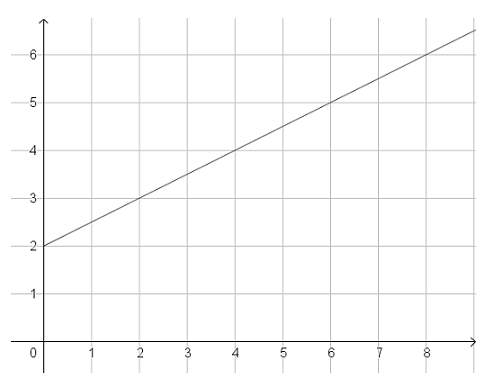# Functions

## Objective

Compare functions represented in different ways (Part 2).

## Common Core Standards

### Core Standards

?

• 8.F.A.2 — Compare properties of two functions each represented in a different way (algebraically, graphically, numerically in tables, or by verbal descriptions). For example, given a linear function represented by a table of values and a linear function represented by an algebraic expression, determine which function has the greater rate of change.

?

• 7.RP.A.2.B

• 7.RP.A.2.C

• 7.RP.A.2.D

## Criteria for Success

?

1. Interpret and compare functions in context of the situation described.
2. Identify rate of change in graphs, tables, equations, and verbal descriptions and compare across representations.
3. Identify initial value in graphs, tables, equations, and verbal descriptions and compare across representations.

## Tips for Teachers

?

• This lesson includes one Anchor Problem, which is longer than a typical Anchor Problem. You may want to allow students to work in pairs or small groups after some independent time with the problem.
• Lesson 10, a continuation from Lesson 9, brings together the concepts and skills from the previous lessons in the unit in order to interpret and compare functions presented in different formats. The EngageNY problems referenced in the Problem Set Guidance include several examples; spread these problems out across Lesson 9 and Lesson 10.

#### Fishtank Plus

• Problem Set
• Student Handout Editor
• Vocabulary Package

## Anchor Problems

?

Sam wants to take his music player and his video game player on a car trip. An hour before they plan to leave, he realized that he forgot to charge the batteries last night. At that point, he plugged in both devices so they can charge as long as possible before they leave.

Sam knows that his music player has 40% of its battery life left and that the battery charges by an additional 12 percentage points every 15 minutes.

His video game player is new, so Sam doesn’t know how fast it is charging but he recorded the battery charge for the first 30 minutes after he plugged it in.

 Time Charging (minutes) 0 10 20 30 Video Game Player Battery Charge (%) 20 32 44 56
1. If Sam’s family leaves as planned, what percent of the battery will be charged for each of the two devices when they leave?
2. How much time would Sam need to charge the battery 100% on both devices?

#### References

Illustrative Mathematics Battery Charging

Battery Charging, accessed on Oct. 31, 2017, 4:05 p.m., is licensed by Illustrative Mathematics under either the CC BY 4.0 or CC BY-NC-SA 4.0. For further information, contact Illustrative Mathematics.

## Problem Set

?The following resources include problems and activities aligned to the objective of the lesson that can be used to create your own problem set.

• Have students represent functions in four ways (tables, equations, graphs, verbal descriptions). If students use notecards to represent the functions, then they can use the notecards in various ways. For example, students could form groups and play Memory with the note cards, turning them over and finding matching pairs. Or, students could make comparisons between the notecards based on rate of change or initial value. The goal of these activities is to enable students to see functions in various representations and to compare across those representations.
• EngageNY Mathematics Grade 8 Mathematics > Module 5 > Topic A > Lesson 7Exercises 1 – 4, Problem Set; use remaining problems not used in Lesson 9; do not include parts of questions where students solve using systems of equations as they have not gotten to that unit yet

?

Function A is shown in the table below.

Function A:

 $x$ 0 2 5 6 8 9 $y$ 2 5 9.5 11 14 15.5

Four more functions are represented below. Find:

• a function that is the same as Function A
• a function with the same rate of change as Function A but with a different initial value
• a function with the same initial value as Function A but with a different rate of change
• a function with a greater rate of change than Function A

Function 1:

During a rainstorm, the water level in a kid-sized pool rises 1.5 inches every hour. Before the rain started, the pool had 1 inch of water in it.

Function 2:

${y=1.5x+2}$

Function 3:Function 4:

 $x$ 0 2 4 6 8 $y$ 0 4 8 12 16

?